Processing ......FreeComputerBooks.com Links to Free Computer, Mathematics, Technical Books all over the World

Analysis and Linear Algebra for Finance
Top Free Python Books 🌠 - 100% Free or Open Source!
• Title Analysis and Linear Algebra for Finance
• Author(s) Patrick Roger
• Publisher: bookboon.com (2013)
• Hardcover/Paperback: N/A
• ebook: PDF Files, Total 283 pages
• Language: English
• ISBN-10: N/A
• ISBN-13: 978-8740303704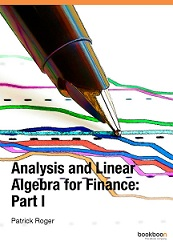Book Description

This book and its companion (part II) present the elements of analysis and linear algebra used in financial models and in microeconomics. Functions of one and several variables and matrices are developed in part I and vector spaces, linear mappings and optimization methods are developed in part II. Instead of formal proofs as in mathematical books, we develop examples and economic illustrations of the use of the concepts presented in the book. The books complement Probability for Finance and Stochastic Processes for Finance providing a broad overview of the mathematics of financial models.

• N/A
Reviews, Ratings, and Recommendations: Related Books and Categories: Read and Download Links:Similar Books:
•Mathematics of Economics and Business (Frank Werner, et al.)

For all students who wish to understand current economic and business literature, knowledge of mathematical methods has become a prerequisite. Clear and concise, with precise definitions and theorems, this book covers all the major topics required.

•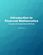Financial Mathematics: Concepts and Computational Methods

This text serves as a primer in financial mathematics with a focus on conceptual understanding of models and problem solving. It includes the mathematical background needed for risk management, such as probability theory, optimization, and the like.

•Financial Mathematics (C.H. Richardson, et al.)

Covering the theories of interest rates, with applications to the evaluation of cash flows, the pricing of fixed income securities and the management of bonds, this textbook also contains numerous examples and exercises of financial calculation.

•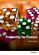Probability for Finance (Patrick Roger)

This book provides technical support for students in finance. It reviews the main probabilistic tools used in financial models in a pedagogical way, starting from simple concepts like random variables and tribes and going to more sophisticated ones like conditional expectations and limit theorems.

•Financial Numerical Recipes in C++: Applications in Finance

This book provides a good deal of useful examples and algorithms for people working within the field of finance, in C++. All the routines have been made to confirm to the new ISO/ANSI C++ standard, using namespaces and the standard template library.

•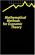Mathematical Methods for Economic Theory (Martin J. Osborne)

This book covers the basic mathematical tools used in economic theory. It emphasizes techniques rather than abstract theory. However, the conditions under which each technique is applicable are stated precisely.

•A Basic Course in the Theory of Interest and Derivatives Markets

This book is designed for an introductory course in the theory of interest and annuity. Each section contains the embedded examples with answer keys. It is suitable for a junior level course in the mathematics of finance.

•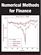Numerical Methods for Finance (Robert Nurnberg)

This book explores new and relevant numerical methods for the solution of practical problems in finance. It is one of the few books entirely devoted to numerical methods as applied to the financial field.

Book Categories
 :All CategoriesTop Free BooksRecent BooksMiscellaneous BooksComputer EngineeringComputer LanguagesComputer ScienceData Science/DatabasesJava and Java EE (J2EE)Linux and UnixMathematicsMicrosoft and .NETMobile ComputingNetworking and CommunicationsSoftware EngineeringSpecial TopicsWeb Programming
Other Categories